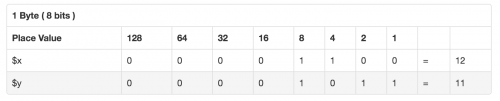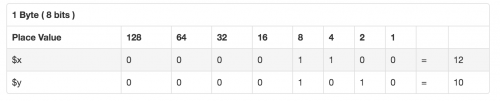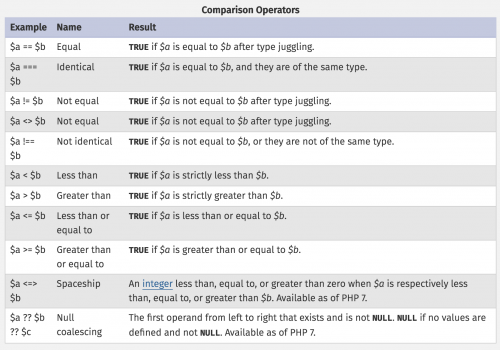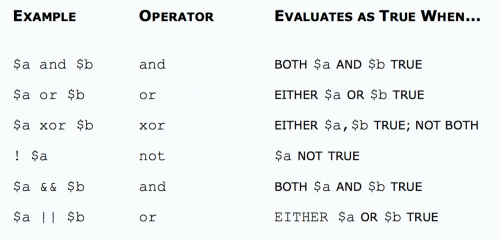# Zend PHP 7 Certification – PHP Basics – Operators

This post covers the Operators section of the PHP Basics chapter when studying for the Zend PHP 7 Certification.

The several types of operators covered are operators commonly used in programming: Arithmetic, Bitwise, Assignment, Comparison, String, Array, Logical, Type and Execution.

## Arithmetic Operators

Like with most programming languages, the basic arithmetic operators are represented by the following characters.

• – -Subtracting)
• * (Multiplying)
• / (Dividing)
• % (Modulus – to calculate the remainder)

There is also the `Exponentiation` arithmetic operator, introduced in PHP 5.6.

``````<?php
\$c = \$a ** \$b; // Result of raising \$a to the \$b'th power``````

Here are some examples of `combined operators` using arithmetic operators. Combined operators can be seen in more detail in the `Assignment Operators` section.

``````<?php
\$a += \$b;  // This is the same as \$a = \$a + \$b
\$a -= \$b;  // This is the same as \$a = \$a - \$b
\$a *= \$b;  // This is the same as \$a = \$a * \$b
\$a /= \$b;  // This is the same as \$a = \$a / \$b
\$a %= \$b;  // This is the same as \$a = \$a % \$b
\$a **= \$b; // This is the same as \$a = \$a ** \$b``````

## Bitwise Operators

Bitwise operators allow evaluation and manipulation of specific bits within an integer. The bitwise operators available are: And, Or (inclusive or), Xor (exclusive or), Not, Shift Left snd Shift Right

Bitwise AND, represented with a `&`, returns the place value present in both variables as represented by the below image.

``````<?php
\$x=13;
\$y=22;
echo \$x & \$y; // Outputs: 4``````

Both values share the bit in third position on place value 4.Bitwise OR, represented with a `|`, returns bits that are set in either \$x or \$y.

``````<?php
\$x=5;
\$y=11;
echo \$x | \$y; // Outputs: 15``````

This is because both values have bits set in the first, second, third and fourth positions.Only the place values are added, therefore 8 + 4 + 2 + 1 = 15.

Bitwise XOR, represented by a `^`, returns bits that are set in \$x or \$y but not in both.

``````<?php
\$x=12;
\$y=11;
echo \$x ^ \$y; // Outputs: 7``````

This is because there is a value set in either \$x or \$yTherefore adding up the place values, 4 + 2 + 1, we get 7.

Bitwise NOT, represented by a `~`, returns bits that are set in \$x but are not set in \$y, and vice vera.

``````<?php
\$x=12;
\$y=10;
echo \$x & ~ \$y; // Outputs: 4

\$x=12;
\$y=10;
echo \$y & ~ \$x; // Outputs: 2``````Bitwise SHIFT LEFT, represented by `<<`, is for scenarios where if \$x and \$y are two numbers, BIT SHIFTING shifts \$a bits by \$b number of steps.

Each step refers to multiply by two if it is BIT SHIFT LEFT. If it is BIT SHIFT RIGHT (represented by `>>`), then each step refers to division by two.

``````<?php
\$x=8;
\$y=3;
echo \$x << \$y; // Outputs: 64. 8 x 2 x 2 x 2 = 64``````

Bitwise SHIFT RIGHT

Shift the bits of \$a \$b steps to the right (each step means “divide by two”)

``````<?php
\$x=8;
\$y=3;
echo \$x >> \$y; // Outputs: 1. 8 / 2 = 4. 4 / 2 = 2. 2 / 2 = 1``````

## Assignment Operators

The most common assignment operator, `=`, sets the left operand to the value on the right. Please note that it does not mean ‘is equal to’ as the character implies.

``\$a = (\$b = 4) + 5; // \$a is set to 9 now, and \$b has been set to 4.``

Assignment by reference means that both variables end up pointing at the same data, and nothing is copied anywhere.

``````\$a = 3;
\$b = &\$a; // \$b is a reference to \$a

echo \$a; // prints 3
echo \$b; // prints 3

\$a = 4; // change \$a to 4

echo \$a; // prints 4
echo \$b; // prints 4 as well, since \$b is a reference to \$a``````

The increment/decrement operators only affect numbers and strings

• `++\$a` – Pre-increment – Increments \$a by one, then returns \$a.
• `\$a++` – Post-increment – Returns \$a, then increments \$a by one.
• `--\$a` – Pre-decrement – Decrements \$a by one, then returns \$a.
• `\$a--` – Post-decrement – Returns \$a, then decrements \$a by one.

## Comparison OperatorsThe spaceship operator returns the following values.

• Returns 0 if values on either side are equal
• Returns 1 if value on the left is greater
• Returns -1 if the value on the right is greater

Examples of this can be seen below.

``````echo 1 <=> 1; // Outputs: 0
echo 3 <=> 4; // Outputs: -1
echo 4 <=> 3; // Outputs: 1

echo "x" <=> "x"; // Outputs: 0
echo "x" <=> "y"; // Outputs: -1
echo "y" <=> "x"; // Outputs: 1``````

## String Operators

The concatenation operator, `.`, returns the concatenation of its right and left arguments.

There is also the concatenating assignment operator, `.=`, which appends the argument on the right side to the argument on the left side.

``````\$a = "Hello ";
\$b = \$a . "World!"; // now \$b contains "Hello World!"

\$a = "Hello ";
\$a .= "World!";     // now \$a contains "Hello World!"``````

## Array Operators

\$a + \$b – Union of \$a and \$b.

Please note that the `+` operator returns the right-hand array appended to the left-hand array. If there are array keys that exist in both arrays, only the elements from the left-hand array will be used. The elements from the right-hand array will be ignored.

``````<?php
\$a = array("a" => "apple", "b" => "banana");
\$b = array("a" => "pear", "b" => "strawberry", "c" => "cherry");

\$c = \$a + \$b; // Union of \$a and \$b
echo "Union of \\$a and \\$b: \n";
var_dump(\$c);

\$c = \$b + \$a; // Union of \$b and \$a
echo "Union of \\$b and \\$a: \n";
var_dump(\$c);

// Union of \$a and \$b:
array(3) {
["a"]=>
string(5) "apple"
["b"]=>
string(6) "banana"
["c"]=>
string(6) "cherry"
}

// Union of \$b and \$a:
array(3) {
["a"]=>
string(4) "pear"
["b"]=>
string(10) "strawberry"
["c"]=>
string(6) "cherry"
}``````

For the first example, the two values apple and banana are taken from \$a and the third value cherry, which only exists in \$b is taken from \$b.

For the second example, the two values pear and strawberry are taken from \$b and the third value cherry, which only exists in \$b is also taken from \$b

\$a == \$b – Equality – TRUE if \$a and \$b have the same key/value pairs

``````\$a = array("apple", "banana");
\$b = array("0" => "apple", "1" => "banana");

var_dump(\$a == \$b); // Outputs: boolean true

\$a = array("apple", "banana");
\$b = array(0 => "apple", 1 => "banana");

var_dump(\$a == \$b); // Outputs: boolean true

\$a = array("apple", "banana");
\$b = array(1 => "apple", 0 => "banana");

var_dump(\$a == \$b); // Outputs: boolean false``````

\$a === \$b – Identity – TRUE if \$a and \$b have the same key/value pairs in the same order and of the same types.

This requires that the keys are in the same order in both arrays.

``````\$a = array("apple", "banana");
\$b = array("1" => "banana", "0" => "apple");

var_dump(\$a == \$b); // Outputs: boolean true

\$a = array("apple", "banana");
\$b = array("1" => "banana", "0" => "apple");

var_dump(\$a === \$b); // Outputs: boolean false``````

\$a != \$b Inequality TRUE if \$a is not equal to \$b.

\$a <> \$b Inequality TRUE if \$a is not equal to \$b.

\$a !== \$b Non-identity TRUE if \$a is not identical to \$b.

## Logical Operators## Type Operators

`instanceof` is used to determine whether a PHP variable is an instantiated object of a certain class.

``````<?php
class MyClass
{
}

class NotMyClass
{
}

\$a = new MyClass;

var_dump(\$a instanceof MyClass); // Outputs: bool(true)
var_dump(\$a instanceof NotMyClass); // Outputs: bool(false)``````

## Execution Operators

Backticks, ````, is the one execution operator that PHP supports. PHP will attempt to execute the contents within the backticks as a shell command.

Use of the backtick operator is identical to running the `shell_exec()` function.

``````<?php

\$output = `ls -al`;
echo "<pre>\$output</pre>";``````

Note that the backtick operator is disabled when safe mode is enabled or if `shell_exec()` is disabled.

View the other sections: